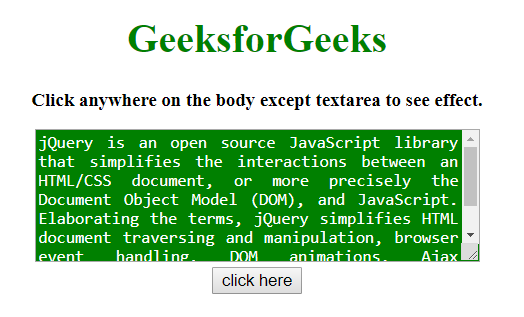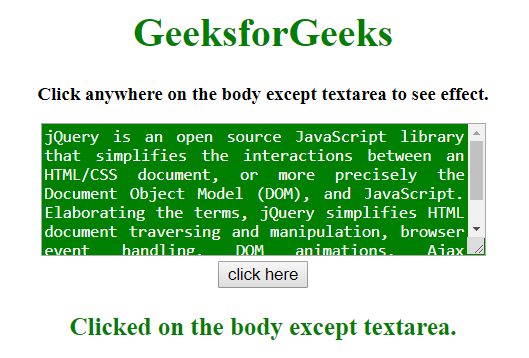# How to click anywhere on the page except one element using jQuery ?

A web-page containing many elements and the task is to click anywhere on the page except one element using jQuery. There are two methods to solve this problem which are discussed below:

Approach 1:

• This approach calls a function when a click event happens.
• First check the id of the targeted element and return the function if it matches.
• Else, perform some operation to let the know that somewhere is clicked.

Example: This example implements the above approach.

 `  ` `<``html``>  ` ` `  `<``head``>  ` `    ``<``title``>  ` `        ``How to click anywhere of page ` `        ``except one element using jQuery ? ` `    `` ` `     `  `    ``<``script` `src``= ` `"https://ajax.googleapis.com/ajax/libs/jquery/3.4.1/jquery.min.js"``> ` `    `` ` `     `  `    ``<``style``> ` `        ``body { ` `            ``height: auto; ` `        ``} ` `        ``#t { ` `            ``height: 100px; ` `            ``width: 350px; ` `            ``background: green; ` `            ``color: white; ` `            ``text-align:justify; ` `        ``} ` `    `` ` `  ` ` `  `<``body` `style` `= ``"text-align:center;"``> ` `     `  `    ``<``h1` `style` `= ``"color:green;"` `>  ` `        ``GeeksforGeeks  ` `    `` ` `     `  `    ``<``p` `id` `= ``"GFG_UP"` `style` `= ` `        ``"font-size: 15px; font-weight: bold;"``> ` `    `` ` `     `  `    ``<``textarea` `id` `= ``"t"``> ` `        ``jQuery is an open source JavaScript library ` `        ``that simplifies the interactions between an ` `        ``HTML/CSS document, or more precisely the  ` `        ``Document Object Model (DOM), and JavaScript. ` `        ``Elaborating the terms, jQuery simplifies ` `        ``HTML document traversing and manipulation, ` `        ``browser event handling, DOM animations,  ` `        ``Ajax interactions, and cross-browser ` `        ``JavaScript development. ` `    `` ` `    ``<``br``> ` `     `  `    ``<``button` `onclick` `= ``"gfg_Run()"``>  ` `        ``click here ` `    `` ` `     `  `    ``<``p` `id` `= ``"GFG_DOWN"` `style = "color:green; ` `        ``font-size: 20px; font-weight: bold;"> ` `    `` ` `     `  `    ``<``script``> ` `        ``var el_up = document.getElementById("GFG_UP"); ` `        ``var el_down = document.getElementById("GFG_DOWN"); ` `         `  `        ``el_up.innerHTML = "Click anywhere on the body " ` `                     ``+ "except textarea to see effect."; ` `         `  `        ``\$('body').click(function(evnt) { ` `             `  `            ``if(evnt.target.id == "t") ` `                ``return; ` `            ``if(\$(evnt.target).closest('t').length) ` `                ``return; ` `             `  `            ``el_down.innerHTML = "Clicked on the " ` `                        ``+ "body except textarea."; ` `        ``}); ` `    ``  ` `  ` ` `  ` `

Output:

• Before clicking on the button:• After clicking on the element:Approach 2:

• This approach calls a function when any click event happens.
• If it is other HTML element then do nothing.
• Else, use event.stopPropagation() method to stop the event from occurring.

Example: This example implements the above approach.

 `  ` `<``html``>  ` ` `  `<``head``>  ` `    ``<``title``>  ` `        ``How to click anywhere of page ` `        ``except one element using jQuery ? ` `    `` ` `     `  `    ``<``script` `src``= ` `"https://ajax.googleapis.com/ajax/libs/jquery/3.4.1/jquery.min.js"``> ` `    `` ` `     `  `    ``<``style``> ` `        ``body { ` `            ``height: auto; ` `        ``} ` `        ``#t { ` `            ``height: 100px; ` `            ``width: 350px; ` `            ``background: green; ` `            ``color: white; ` `            ``text-align:justify; ` `        ``} ` `    `` ` `  ` ` `  `<``body` `style` `= ``"text-align:center;"``> ` `     `  `    ``<``h1` `style` `= ``"color:green;"` `>  ` `        ``GeeksforGeeks  ` `    `` ` `     `  `    ``<``p` `id` `= ``"GFG_UP"` `style` `= ` `        ``"font-size: 15px; font-weight: bold;"``> ` `    `` ` `     `  `    ``<``textarea` `id` `= ``"t"``> ` `        ``jQuery is an open source JavaScript library ` `        ``that simplifies the interactions between an ` `        ``HTML/CSS document, or more precisely the  ` `        ``Document Object Model (DOM), and JavaScript. ` `        ``Elaborating the terms, jQuery simplifies ` `        ``HTML document traversing and manipulation, ` `        ``browser event handling, DOM animations,  ` `        ``Ajax interactions, and cross-browser ` `        ``JavaScript development. ` `    `` ` `    ``<``br``> ` `     `  `    ``<``button` `onclick` `= ``"gfg_Run()"``>  ` `        ``click here ` `    `` ` `     `  `    ``<``p` `id` `= ``"GFG_DOWN"` `style = "color:green; ` `        ``font-size: 20px; font-weight: bold;"> ` `    `` ` `     `  `    ``<``script``> ` `        ``var el_up = document.getElementById("GFG_UP"); ` `        ``var el_down = document.getElementById("GFG_DOWN"); ` `         `  `        ``el_up.innerHTML =  "Click anywhere on the body" ` `                    ``+ " except textarea to see effect."; ` `         `  `        ``\$('html').click(function() { ` `            ``el_down.innerHTML = "Clicked on the body" ` `                        ``+ " except textarea."; ` `        ``}); ` `         `  `        ``\$('#t').click(function(event) { ` `            ``event.stopPropagation(); ` `        ``}); ` `    ``  ` `  ` ` `  ` `

Output:

• Before clicking on the button:• After clicking on the element:My Personal Notes arrow_drop_upCheck out this Author's contributed articles.

If you like GeeksforGeeks and would like to contribute, you can also write an article using contribute.geeksforgeeks.org or mail your article to contribute@geeksforgeeks.org. See your article appearing on the GeeksforGeeks main page and help other Geeks.

Please Improve this article if you find anything incorrect by clicking on the "Improve Article" button below.